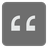Sum Numbers
Elementary
English PL RU

In a given text you need to sum the numbers. Only separated numbers should be counted. If a number is part of a word it shouldn't be counted.

The text consists from numbers, spaces and english letters

Input: A string.

Output: An int.

Example:

```sum_numbers('hi') == 0
sum_numbers('who is 1st here') == 0
sum_numbers('my numbers is 2') == 2
sum_numbers('This picture is an oil on canvas '
'painting by Danish artist Anna '
'Petersen between 1845 and 1910 year') == 3755
sum_numbers('5 plus 6 is') == 11
sum_numbers('') == 0
```